Need help? Email help@shout.education

# Iron

This page looks at some aspects of iron chemistry required for UK A-level exams (summarised from elsewhere on the site). You will find links to pages where full details and explanations are given.

## Iron and its Ions as Aatalysts

### Iron as Catalyst in the Haber Process

The Haber Process combines nitrogen and hydrogen into ammonia. The nitrogen comes from the air and the hydrogen is obtained mainly from natural gas (methane). Iron is used as a catalyst.

\text{N}_{2(g)} + 3\text{H}_{2(g)} \xrightleftharpoons{\text{Fe}} 2\text{NH}_{3(g)}

Note: You can find a full discussion about the Haber Process by following this link.

### Iron Ions as a Catalyst in the Reaction Between Persulfate Ions and Iodide Ions

The reaction between persulfate ions (peroxodisulfate ions), S2O82-, and iodide ions in solution can be catalysed using either iron(II) or iron(III) ions.

The overall equation for the reaction is:

{\text{S}_2\text{O}_8}^{2-} + 2\text{I}^- \longrightarrow 2{\text{SO}_4}^{2-} + \text{I}_2

For the sake of argument, we'll take the catalyst to be iron(II) ions. The reaction happens in two stages.

\begin{gathered} {\text{S}_2\text{O}_8}^{2-} + 2\text{Fe}^{2+} \longrightarrow 2{\text{SO}_4}^{2-} + 2\text{Fe}^{3+} \\ \\ 2\text{Fe}^{3+} + 2\text{I}^- \longrightarrow 2\text{Fe}^{2+} + \text{I}_2 \end{gathered}

If you use iron(III) ions, the second of these reactions happens first.

This is a good example of the use of transition metal compounds as catalysts because of their ability to change oxidation state.

Note: This reaction is explained in more detail on a page about types of catalysis.

## Reactions of Iron Ions in Solution

The simplest ions in solution are:

• the hexaaquairon(II) ion – [Fe(H2O)6]2+.
• the hexaaquairon(III) ion – [Fe(H2O)6]3+.

They are both acidic, but the iron(III) ion is more strongly acidic.

Note: If you aren't happy about complex ions (including the way they are bonded and named), it would pay you to follow this link and explore the first couple of pages in the complex ions menu before you go on.

The acidity of the complex ions is discussed in detail on another page.

### Reactions of the Iron Ions With Hydroxide Ions

Hydroxide ions (from, say, sodium hydroxide solution) remove hydrogen ions from the water ligands attached to the iron ions.

When enough hydrogen ions have been removed, you are left with a complex with no charge – a neutral complex. This is insoluble in water and a precipitate is formed.

##### In the iron(II) case:
[\text{Fe}(\text{H}_2\text{O})_6]^{2+} + \color{#467abf}{2\text{OH}^-} \longrightarrow [\text{Fe}(\text{H}_2\text{O})_4(\text{OH})_2] + \color{#467abf}{2\text{H}_2\text{O}}

In the iron(III) case:

[\text{Fe}(\text{H}_2\text{O})_6]^{3+} + \color{#467abf}{3\text{OH}^-} \longrightarrow [\text{Fe}(\text{H}_2\text{O})_3(\text{OH})_3] + \color{#467abf}{3\text{H}_2\text{O}}

Note: The colour coding is to show that these aren't ligand exchange reactions. The oxygens which were originally attached to the iron are still attached in the neutral complexes.

##### In the iron(II) case: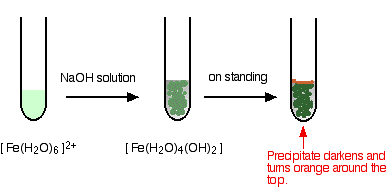Iron is very easily oxidised under alkaline conditions. Oxygen in the air oxidises the iron(II) hydroxide precipitate to iron(III) hydroxide especially around the top of the tube. The darkening of the precipitate comes from the same effect.

##### In the iron(III) case: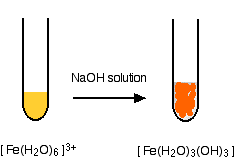Note: You will find the reactions between hexaaqua ions and hydroxide ions discussed in detail if you follow this link.

### Reactions of the Iron Ions With Ammonia Solution

Ammonia can act as both a base and a ligand. In these cases, it simply acts as a base – removing hydrogen ions from the aqua complex.

##### In the iron(II) case:
[\text{Fe}(\text{H}_2\text{O})_6]^{2+} + 2\text{NH}_3 \longrightarrow [\text{Fe}(\text{H}_2\text{O})(\text{OH})_2] + 2{\text{NH}_4}^+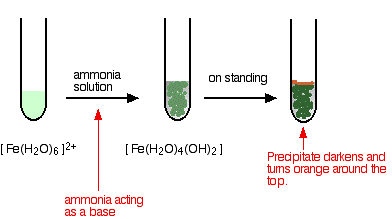The appearance is just the same as in when you add sodium hydroxide solution. The precipitate again changes colour as the iron(II) hydroxide complex is oxidised by the air to iron(III) hydroxide.

##### In the iron(III) case:
[\text{Fe}(\text{H}_2\text{O})_6]^{3+} + 3\text{NH}_3 \longrightarrow [\text{Fe}(\text{H}_2\text{O})(\text{OH})_3] + 3{\text{NH}_4}^+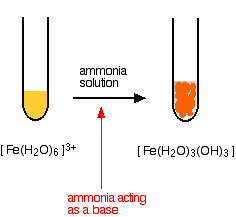The reaction looks just the same as when you add sodium hydroxide solution.

Note: You will find the reactions between hexaaqua ions and ammonia solution discussed in detail if you follow this link.

### Reactions of the Iron Ions With Carbonate Ions

There is an important difference here between the behaviour of iron(II) and iron(III) ions.

#### Iron(II) ions and carbonate ions

You simply get a precipitate of what you can think of as iron(II) carbonate.

\text{Fe}^{2+}_{(aq)} + {\text{CO}_3}^{2-}_{(aq)} \longrightarrow \text{FeCO}_{3(s)}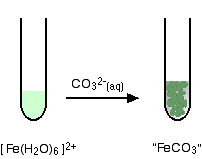Note: Only one of the UK A-level Exam Boards wants this, and this is the simplification that they make. In fact, the precipitate is better described as a basic carbonate with a formula of the type xFeCO3,yFe(OH)2,zH2O.

#### Iron(III) ions and carbonate ions

The hexaaquairon(III) ion is sufficiently acidic to react with the weakly basic carbonate ion.

If you add sodium carbonate solution to a solution of hexaaquairon(III) ions, you get exactly the same precipitate as if you added sodium hydroxide solution or ammonia solution.

This time, it is the carbonate ions which remove hydrogen ions from the hexaaqua ion and produce the neutral complex.

Depending on the proportions of carbonate ions to hexaaqua ions, you will get either hydrogencarbonate ions formed or carbon dioxide gas from the reaction between the hydrogen ions and carbonate ions. The more usually quoted equation shows the formation of carbon dioxide.

2[\text{Fe}(\text{H}_2\text{O})_6]^{3+} + 3{\text{CO}_3}^{2-} \longrightarrow 2[\text{Fe}(\text{H}_2\text{O})_3(\text{OH})_3] + 3\text{CO}_2 + 3\text{H}_2\text{O}

Apart from the carbon dioxide, there is nothing new in this reaction: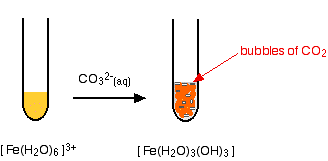Note: You will find the reactions between hexaaqua ions and carbonate ions discussed in detail if you follow this link.

### Testing for Iron(III) Ions With Thiocyanate Ions

This provides an extremely sensitive test for iron(III) ions in solution.

If you add thiocyanate ions, SCN-, (from, say, sodium or potassium or ammonium thiocyanate solution) to a solution containing iron(III) ions, you get an intense blood red solution containing the ion [Fe(SCN)(H2O)5]2+.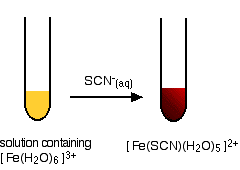Note: I haven't been able to suggest properly the intense rich red of the thiocyano complex. Imagine blood!

This is a ligand exchange reaction. You can find out more about these by following this link.

### Finding the Concentration of Iron(II) Ions in Solution by Titration

You can find the concentration of iron(II) ions in solution by titrating with either potassium manganate(VII) solution or potassium dichromate(VI) solution. The reactions are done in the presence of dilute sulfuric acid.

In either case, you would pipette a known volume of solution containing the iron(II) ions into a flask, and add a roughly equal volume of dilute sulfuric acid. What happens next depends on whether you are using potassium manganate(VII) solution or potassium dichromate(VI) solution.

#### Using potassium manganate(VII) solution

The potassium manganate(VII) solution is run in from a burette. At first, it turns colourless as it reacts. The end point is the first trace of permanent pink in the solution showing a tiny excess of manganate(VII) ions.

The manganate(VII) ions oxidise iron(II) to iron(III) ions. The two half-equations for the reaction are:

\begin{aligned} \text{Fe}^{2+} &\longrightarrow \text{Fe}^{3+} + \text{e}^- \\ \\ {\text{MnO}_4}^- + 8\text{H}^+ + 5\text{e}^- &\longrightarrow \text{Mn}^{2+} + 4\text{H}_2\text{O} \end{aligned}

These combine to give the ionic equation for the reaction:

{\text{MnO}_4}^- + 8\text{H}^+ + 5\text{Fe}^{2+} \longrightarrow \text{Mn}^{2+} + 4\text{H}_2\text{O} + 5\text{Fe}^{3+}

Note: If you don't know how to work out half-equations and combine them into equations like this, you can find out how to do it on the page about writing ionic equations for redox reactions.

The complete equation shows that 1 mole of manganate(VII) ions react with 5 moles of iron(II) ions. Having got that information, the titration calculations are just like any other ones.

Note: If you aren't very good at titration calculations, you might be interested in my chemistry calculations book.

There is more about potassium manganate(VII) titrations on the page about manganese chemistry.

#### Using potassium dichromate(VI) solution

Potassium dichromate(VI) solution turns green as it reacts with the iron(II) ions, and there is no way you could possibly detect the colour change when you have one drop of excess orange solution in a strongly coloured green solution.

With potassium dichromate(VI) solution you have to use a separate indicator, known as a redox indicator. These change colour in the presence of an oxidising agent.

There are several such indicators – such as diphenylamine sulfonate. This gives a violet-blue colour in the presence of excess potassium dichromate(VI) solution.

##### The two half-equations are:
\begin{aligned} \text{Fe}^{2+} &\longrightarrow \text{Fe}^{3+} + \text{e}^- \\ \\ {\text{Cr}_2\text{O}_7}^{2-} + 14\text{H}^+ + 6\text{e}^- &\longrightarrow 2\text{Cr}^{3+} + 7\text{H}_2\text{O} \end{aligned}
##### Combining these gives:
{\text{Cr}_2\text{O}_7}^{2-} + 14\text{H}^+ + 6\text{Fe}^{2+} \longrightarrow 2\text{Cr}^{3+} + 7\text{H}_2\text{O} + 6\text{Fe}^{3+}

You can see that the reacting proportions are 1 mole of dichromate(VI) ions to 6 moles of iron(II) ions.

Once you have established that, the titration calculation is again going to be just like any other one.

Note: There is more about potassium dichromate(VI) titrations on the page about chromium chemistry.